## Vito Corleone has planned a grand outdoor summer wedding for his daughter in New York City. In the past couple of years, it has rained only

Question

Vito Corleone has planned a grand outdoor summer wedding for his daughter in New York City. In the past couple of years, it has rained only 25 days out of the 92 days of summer. Unfortunately for Vito, the local meteorologist has predicted that it will rain on the day of the wedding. On a typical summer day: given it rains, the meteorologist predicted that it will rain correctly) 80% of the time On the other hand, given it did not rain, the meteorologist predicted it will rain (incorrectly 20% of the time.
A. What is the probability that the meteorologist predicts that it rains on a given summer day?
B. Given that the meteorologist predicted rain, what is the probability that it actually rains on the day of the wedding?

in progress 0
6 months 2021-07-22T02:48:33+00:00 1 Answers 0 views 0

a) 0.3630 = 36.30% probability that the meteorologist predicts that it rains on a given summer day.

b) 0.5989 = 59.89% probability that it actually rains on the day of the wedding

Step-by-step explanation:

Conditional Probability

We use the conditional probability formula to solve this question. It is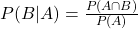In which

P(B|A) is the probability of event B happening, given that A happened.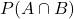is the probability of both A and B happening.

P(A) is the probability of A happening.

A. What is the probability that the meteorologist predicts that it rains on a given summer day?

80% of 25/92(prediction of rain, and rained).

20% of 67/92(prediction of rain, did not rain). So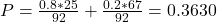0.3630 = 36.30% probability that the meteorologist predicts that it rains on a given summer day.

B. Given that the meteorologist predicted rain, what is the probability that it actually rains on the day of the wedding?

Conditional Probability, in which:

Event A: prediction of rain

Event B: rain

0.3630 = 36.30% probability that the meteorologist predicts that it rains on a given summer day, which means that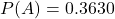Rain and prediction of rain:

80% of 25/92. So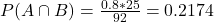The desired probability is: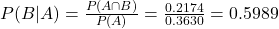0.5989 = 59.89% probability that it actually rains on the day of the wedding